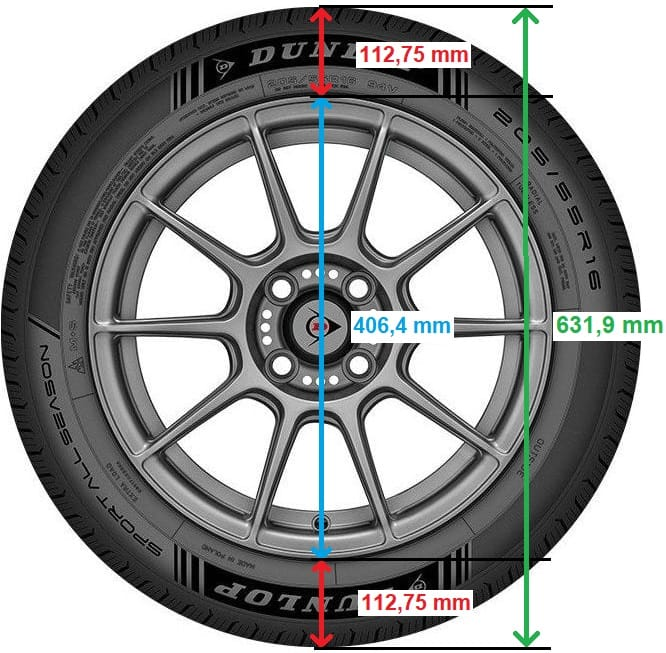# Rounding circumference calculator

Subjects:

• Calculator
• Tire height and static rolling circumference
• Calculation example

calculator:
Placing the wrong tire size under the car will result in a deviation on the odometer. The exact amount of the deviation can be calculated in the calculator below. Click on the image to open the calculator in a new screen. At the top of the table, enter the tire size that belongs under the car (which now reads 205/55R16) and below that the tire size that you want to know if it is suitable for mounting.

Tire height and static unwinding circumference:
The static tire height and rolling circumference can be calculated in the calculator. This is ideal when looking for a different tire size (e.g. changing from 16 to 18 inch) that can fit under the car without affecting the speedometer display.

The tire in the example is marked: 205/55 R16:

• 205 = tread width in mm
• 55 = tire height from road surface to underside of rim = 55% of 205 (0.55 x 205 = 112.75 mm)
• 16 = rim diameter in inches (1 inch = 25.4 mm, so 16 inch x 25.4 mm = 406.4 mm)

Enter these 3 values ​​in the calculator to calculate the tire height and the static rolling circumference in an unloaded condition. The tire height is twice the height in millimetres, plus the rim diameter in millimetres. So in the example above: 112,75 + 112,75 + 406,4 = 631,9 mm (or 63,19 cm). In this calculator you can easily calculate the tire heights of different tire sizes and the difference in circumference and speed.The two tire sizes that are standard in the table (the 205/55R16 and the 225/40R18) have a very small difference in height and circumference. As a result, the speed difference on the odometer is also minimal. This means that if the size 205/55R16 is specified by the manufacturer, wheels with tire size 225/40R18 can also be fitted. The only question then is whether the wider tires will not run into the wheel arches. If this is the case when you send it in, it can cause a lot of wear to the tire and that is a reason for rejection at the MOT. In that case, track wideners offer a solution.